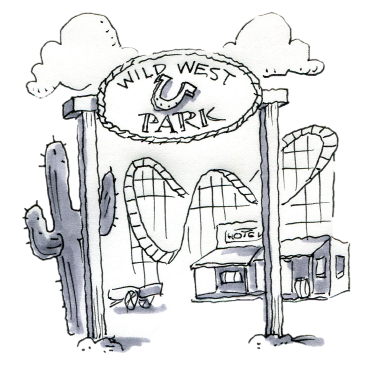### Home > CC3MN > Chapter 9 > Lesson 9.1.2 > Problem9-26

9-26.

The Wild West Frontier Park now offers an unlimited day pass.  For $29.00$, visitors can go on as many rides as they want.  The original plan charged visitors $8.75$ to enter the park, plus $2.25$ for each ride.  Write an equation to determine the number of rides that would make the total cost equal for the two plans.  Solve the equation.Create a variable expression to represent this problem.
Use the number of rides as a variable.

Set the two parts of the equation equal to each other to find the value of the variable, or the number of rides, where both plans would have equal costs.

Consider $8.75$ as a constant and that each ride costs $2.25$ when writing your equation.

$29 = 8.75 + 2.25x$
$x = 9 \text{ rides}$